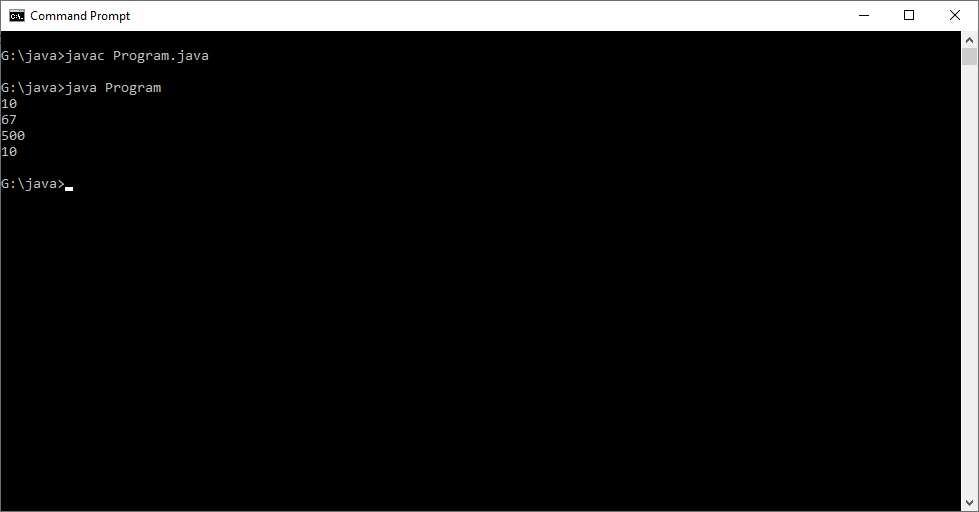# Implicit Type Conversion Example in Java

Write a program to perform implicit type conversion

#### Program or Solution

``` class Program{ public static void main(String args[]) { byte b = 10; char ch = 'C'; short num = 500; int i; i = b; System.out.println(i); i = ch; System.out.println(i); i = num; System.out.println(i); num = b; System.out.println(num); //Following Conversions are not possible implictly because size of i is 4 bytes, ch is 2 bytes, b is 1 byte and num is 2 bytes  //b=i; // size of i is greater than b //ch =i; // size of i is greater than ch //b = num; // size of num is greater than b //num = i; // size of i is greater than num }} ```

#### Output#### Program Explanation

Implicit Type casting take place when, the two types are compatible, the target type is larger than the source type.

The Commented lines are not possible because source types are larger than destination types.